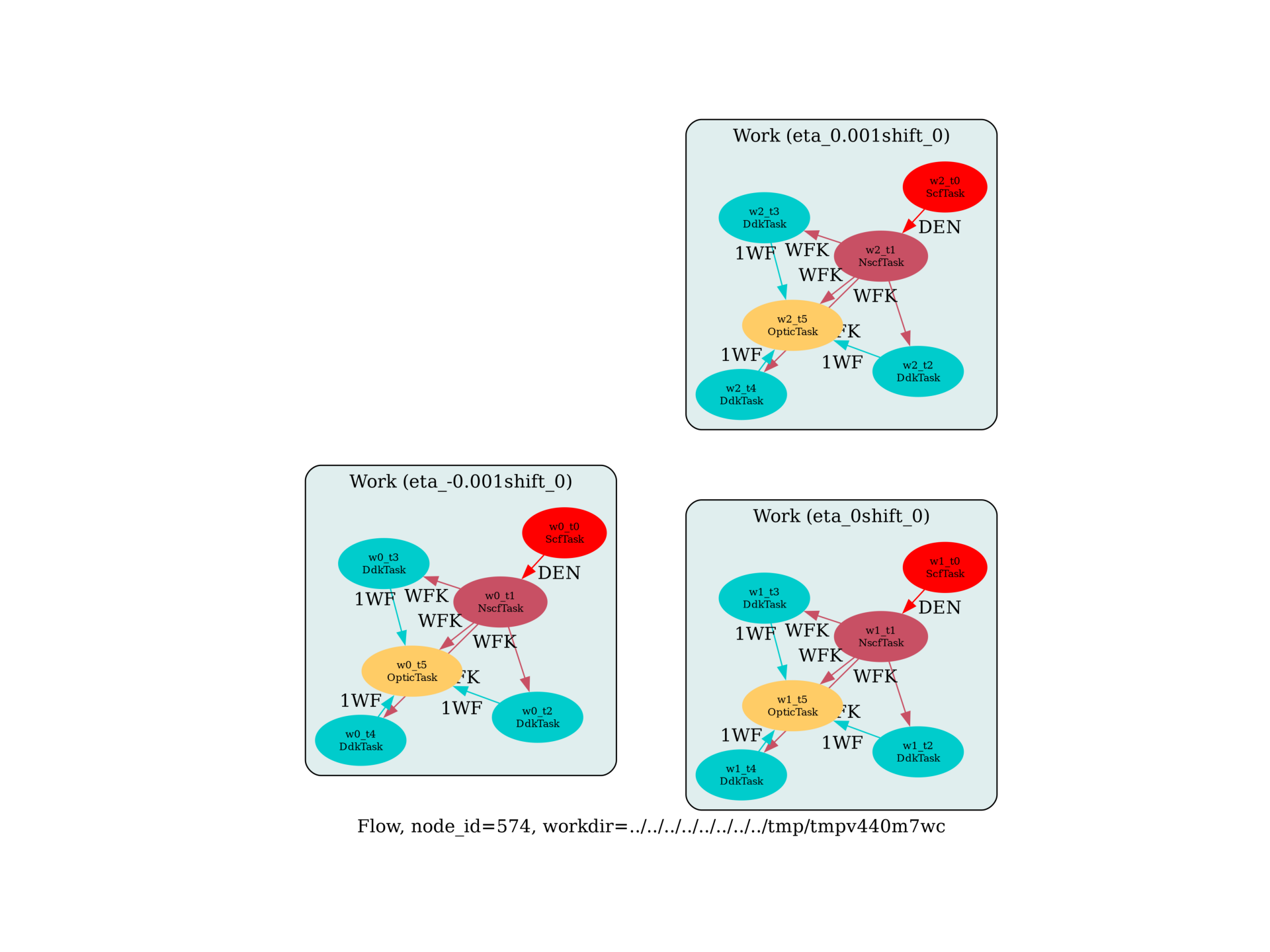Raman Flow with independent-particle approximation (optic)¶

This script shows how to perform a Raman calculation with excitonic effects included with the BSE formalism.import sys
import os
import numpy as np

import abipy.abilab as abilab
import abipy.data as abidata
import abipy.flowtk as flowtk

def build_flow(options):
# Get the unperturbed structure.
pseudos = abidata.pseudos("14si.pspnc")
base_structure = abidata.structure_from_ucell("Si")

ngkpt = [6, 6, 6]

etas = [-.001, 0, +.001]
ph_displ = np.reshape(np.zeros(3*len(base_structure)), (-1,3))
ph_displ[0,:] = [+1, 0, 0]
ph_displ[1,:] = [-1, 0, 0]

# Build new structures by displacing atoms according to the phonon displacement
# ph_displ (in cartesian coordinates). The Displacement is normalized so that
# the maximum atomic diplacement is 1 Angstrom and then multiplied by eta.
modifier = abilab.StructureModifier(base_structure)

displaced_structures = modifier.displace(ph_displ, etas, frac_coords=False)

# Initialize flow. Each workflow in the flow defines a complete BSE calculation for given eta.
if not options.workdir:
options.workdir = os.path.basename(sys.argv).replace(".py", "").replace("run_", "flow_")

flow = flowtk.Flow(options.workdir, manager=options.manager)

# Generate the different shifts to average
ndiv = 1
shift1D = np.arange(1, 2*ndiv+1, 2) / (2 * ndiv)
all_shifts = [[x, y, z] for x in shift1D for y in shift1D for z in shift1D]

for structure, eta in zip(displaced_structures, etas):
for ishift,shift in enumerate(all_shifts):
flow.register_work(raman_work(structure, pseudos, ngkpt, shift),
workdir="eta_" + str(eta) + "shift_" + str(ishift))

return flow

def raman_work(structure, pseudos, ngkpt, shiftk):
# Generate 3 different input files for computing optical properties with Optic.

global_vars = dict(
istwfk="*1",
paral_kgb=0,
ecut=8,
nstep=200,
diemac=12,
ixc=7,
chksymbreak=0,
#accesswff=3
)

multi = abilab.MultiDataset(structure, pseudos=pseudos, ndtset=5)
multi.set_vars(global_vars)
multi.set_kmesh(ngkpt=ngkpt, shiftk=shiftk)

# GS run
multi.set_vars(
tolvrs=1e-8,
nband=20,
nbdbuf=2,
)

# Note kptopt 2 in NSCF and DDK
# In principle kptopt 2 is needed only in DDK.
# one could do a first NSCF run with kptopt 1, reread with kptopt 2 and enter DDK...

# NSCF run
multi.set_vars(
iscf=-2,
nband=40,
nbdbuf=5,
kptopt=2,
tolwfr=1.e-12,
)

# DDK along 3 directions
# Third dataset : ddk response function along axis 1
# Fourth dataset : ddk response function along axis 2
# Fifth dataset : ddk response function along axis 3
for idir in range(3):
rfdir = 3 * 
rfdir[idir] = 1

multi[2 + idir].set_vars(
iscf=-3,
nband=40,
nbdbuf=5,
nstep=1,
nline=0,
prtwf=3,
kptopt=2,
nqpt=1,
qpt=[0.0, 0.0, 0.0],
rfdir=rfdir,
rfelfd=2,
tolwfr=1.e-12,
)

scf_inp, nscf_inp, ddk1, ddk2, ddk3 = multi.split_datasets()
ddk_inputs = [ddk1, ddk2, ddk3]

work = flowtk.Work()

ddk_nodes = []
for inp in ddk_inputs:
ddk_nodes.append(ddk_t)

optic_input = abilab.OpticInput(
broadening=0.002,     # Value of the smearing factor, in Hartree
domega=0.0003,        # Frequency mesh.
maxomega=0.3,
scissor=0.000,        # Scissor shift if needed, in Hartree
tolerance=0.002,      # Tolerance on closeness of singularities (in Hartree)
num_lin_comp=6,       # Number of components of linear optic tensor to be computed
lin_comp=(11, 12, 13, 22, 23, 33), # Linear coefficients to be computed (x=1, y=2, z=3)
num_nonlin_comp=0     # Number of components of nonlinear optic tensor to be computed
#nonlin_comp=(123, 222),
)

work.register(optic_t)

return work

# This block generates the thumbnails in the AbiPy gallery.
# You can safely REMOVE this part if you are using this script for production runs.
__name__ = None
import tempfile
options = flowtk.build_flow_main_parser().parse_args(["-w", tempfile.mkdtemp()])
build_flow(options).graphviz_imshow()

@flowtk.flow_main
def main(options):
"""
This is our main function that will be invoked by the script.
flow_main is a decorator implementing the command line interface.
Command line args are stored in `options`.
"""
return build_flow(options)

if __name__ == "__main__":
sys.exit(main())

Total running time of the script: ( 0 minutes 0.822 seconds)

Gallery generated by Sphinx-Gallery